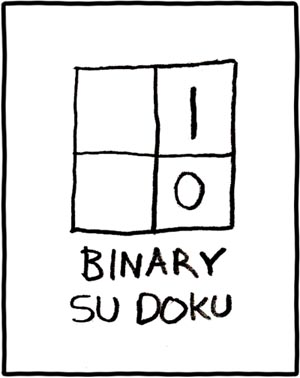# Two Guys Arguing

## A Solution from Purgatory (for the Matrix from Hell)

Posted in challenge, math, questions by benjaminplee on 01.02.11

###This post attempts to recount some of my thought process which eventually (more than a year later) lead to the Matrix from Hell puzzle I posted about a few days ago.  If you don’t want to know my answer, don’t read the rest of this post.  My final solution is at the bottom.

#### You have been warned.

~~~~~~~~~~~~~~~~~~~

~~~~~~~~~~~~~~~~~~~~~~~~~~~~~~~~~~~~~~

~~~~~~~~~~~~~~~~~~~

Below is a list of my general thought process and the main ideas/revelations/halucinations that I know helped me to my solution.  An annotated matrix is at the bottom with some of the ideas highlighted in different colors.

• Should indices start at 0 or 1?
• Values along either axis count up by 1 in both directions when the other =0
• Values are duplicated over the f(x)=y axis –> Order of indices given to function is inmaterial since they can be swapped
[The RED 5 can be seen duplicated over the diagonal]
• Calculation of max/min index is O(1) so stop worry about it and “forget” the bottom half of the matrix (assume X>= Y)
• Values generally increase as they go right/down but not always
• f(x,x)=0, f(x,0)=x
• Values are symmetrical perpendicular to f(x)=y IFF the bounding square in question is a power of 2
• “Quad” patterns can be seen repeating for every power of 2.  In each “Quad” the top left quadrant is the same as the bottom right and the top right is the same as the bottom left.
[Each “Quad” of the example matrix is outlined in BLUE with the quadrants in LIGHT BLUE. PINK shows the repetition]
• power of 2 repeating nature of the values leads to thinking of binary trees
• binary trees leads to thinking about a possible solution which could run in O(log2 n) time:
• In a nut shell, for a given (X,Y), algorithm would find smallest boudning power of 2 including X and would walk back “Quad”s by each successivly smaller power of 2 “Quad” until the last one is met.  For each “Quad” determine which quadrant the value lives in and remap to root values.
• Debate if this would really work for large numbers and if it could be reduced to constant time function by math that I have forgotton from college/HS
• Dismiss b/c I can’t remember a way and base idea is > O(1)
• Binary trace back algorithm idea makes me realize that it can be also looked at as the “binary” representation of a number (e.g. 01011011010) where each 1 represents a “Quad” where the value was in the upper right or bottom left and vica-verca.
[Following the matrix value at (5,6) can be seen ORANGE.  The value 3 is in the bottom right  of the 1st “Quad”, then BL, & TR. {BR BL TR} => {101} => {3}]
• Remembered that f(x,y)=0 IFF x==y …. which means that difference between X and Y is important to value of f()
• Eureka!
• F(X, Y) = X xor Y
• solution typed above in white on white to protect the innocent. highlight to view.

### That is it.  I never said it was pretty or made any sense.

Tagged with: , , , ,

### One Response

1.Peter de Rivaz said, on 07.19.13 at 8:50 am

By the way, you can also view this Matrix puzzle as a 2 player game where you can move left any number of squares, or up any number of squares. The winner is the first player to reach the top left.

This game is also isomorphic to a 2 stack game of Nim.

The general approach to computing who wins such games is to compute the Nim-numbers of the game. A nim-number is computed as the smallest non-negative number not already present in any position you can move to.

This operation of finding the smallest non-negative is sometimes called the “mex” function.

The numbers in your matrix are precisely the nim-numbers of this game.

It is well known in game theory that you can compute the Nim number of multiple stacks of Nim by XORing the sizes of the piles. (Although this is the first time I’ve encountered someone who has managed to work out the formula by themselves!)

In summary, this is another way to see why your solution is correct that you might find interesting.

Note also that your formula would work just as well for a 3d matrix (the function becomes x^y^z).